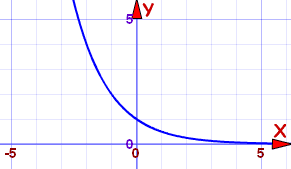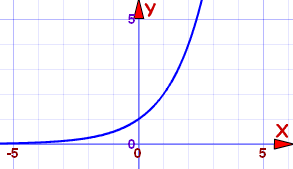# Exponential Function Reference

This is the general Exponential Function (see below for ex):

f(x) = ax

a is any value greater than 0

### Properties depend on value of "a"

• When a=1, the graph is a horizontal line at y=1
• Apart from that there are two cases to look at:

a between 0 and 1Example: f(x) = (0.5)x

For a between 0 and 1

a above 1Example: f(x) = (2)x

For a above 1:

Plot the graph here (use the "a" slider)

### In General:

• It is always greater than 0, and never crosses the x-axis
• It always intersects the y-axis at y=1 ... in other words it passes through (0,1)
• At x=1, f(x)=a ... in other words it passes through (1,a)
• It is an Injective (one-to-one) function

Its Domain is the Real Numbers:Its Range is the Positive Real Numbers: (0, +∞)

## Inverse

ax   is the inverse function of   loga(x) (the Logarithmic Function)

So the Exponential Function can be "reversed" by the Logarithmic Function.

## The Natural Exponential Function

This is the "Natural" Exponential Function:

f(x) = ex

Where e is "Eulers Number" = 2.718281828459... etcGraph of f(x) = ex

The value e is important because it creates these useful properties:

At any point the slope of ex equals the value of ex :when x=0, the value of ex = 1, and slope = 1
when x=1, the value of ex = e, and slope = e
etc...

The area up to any x-value is also equal to ex :